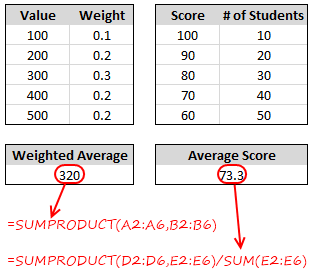## Weighted Average in Excel [Formulas]

Today we will learn how to calculate weighted averages in excel using formulas.

### What is weighted average ?

Weighted average or weighted mean is defined as [from wikipedia],

The weighted mean is similar to an arithmetic mean …, where instead of each of the data points contributing equally to the final average, some data points contribute more than others.

If all the weights are equal, then the weighted mean is the same as the arithmetic mean.

### Why should you calculate weighted average?

or in other words, why you should  not calculate normal average?

Well, it is because, in some situations normal averages give in-correct picture. For eg. assume you are the CEO of ACME Widgets co.. Now you are looking annual salary report and being the numbers-gal you are, you wanted to find-out the average salary of your employees. You asked each department head to give you the average salary of that department to you. Here are the numbers,Now, the average salary seems to be \$ 330,000 [total all of all salaries by 5, (55000+65000+75000+120000+1200000)/5 ].

You are a happy boss to find that your employees are making \$330k per year.

Except, you are wrong. You have not considered the number of employees in each department before calculating the average. So, the correct average would be \$76k as shown above.

### How to calculate weighted average in Excel?There is no built-in formula in Excel to calculate weighted averages. However, there is an easy fix to that. You can use SUMPRODUCT formula. By definition, SUMPRODUCT formula takes 2 or more lists of numbers and returns the sum of product of corresponding values. [related: Excel SUMPRODUCT Formula – what is it and how to use it?]

So, if you have values in A1:A10 and the corresponding weights in B1:B10, you can use SUMPRODUCT like this to get weighted average.

`=SUMPRODUCT(A1:A10,B1:B10)`

However, the above method works only if B1:B10 contains weights in %s totaling to 100%.

In the above average salary example, we dont have weights. But we have count of values. This is when you can use a variation of SUMPRODUCT Formula like this:

`=SUMPRODUCT(A1:A10,B1:B10)/SUM(B1:B10)`

In this workbook, you can find 3 examples on how to to calculate weighted average in excel. Go ahead and download it to understand the formulas better.

### Weighted Average in Excel – Formula Explained

Here is a video with Weighted Average formula explained. Please watch it below to learn more. Alternatively, head to my YouTube page to see the weighted averages in Excel video.

### Do you use Weighted Mean / Weighted Average?

What do you use it for? What kind of challenges you face? Do you apply any tweaks to weighted average calculations? Please share your ideas / tips using comments.

### More examples on Averages and Formulas:

• Syntax and Examples of Excel Average Formula
• Formula for Average of Top 5 values
• Calculating Moving Average in Excel
• Using SUBTOTAL formula and calculating averages
• Showing Averages in Pivot Tables

The post Weighted Average in Excel [Formulas] appeared first on Chandoo.org – Learn Excel, Power BI & Charting Online.